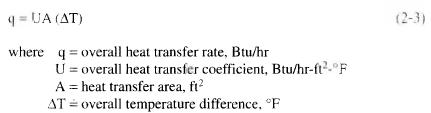﻿ Multiple Transfer Mechanisms | Oil & Gas Process Engineering

## Multiple Transfer Mechanisms

Most heat transfer processes used in production facilities involve combinations of conduction and convection transfer processes. For example, in heat exchangers the transfer of heat energy from the hot fluid to the cold fluid involves three steps. First, the heat energy is transferred from the hot fluid to the exchanger tube, then through the exchanger tube wall, and finally from the tube wall to the cold fluid. The first and third steps are convection transfer processes, while the second step is conduction process,

To calculate the rate of heat transfer in each of the steps, the individual temperature difference would have to be known. It is difficult to measure accurately the temperatures at each boundary, such as at the surface of the heat exchanger tube. Therefore, in practice, the heat transfer calculations are based on the overall temperature difference, such as the difference between the hot and cold fluid temperatures. The heat transfer rate is expressed by the following equation, similar to the conductive/convective transfer process:Examples of overall heat transfer coefficient and overall temperature difference calculations are discussed in the following sections.